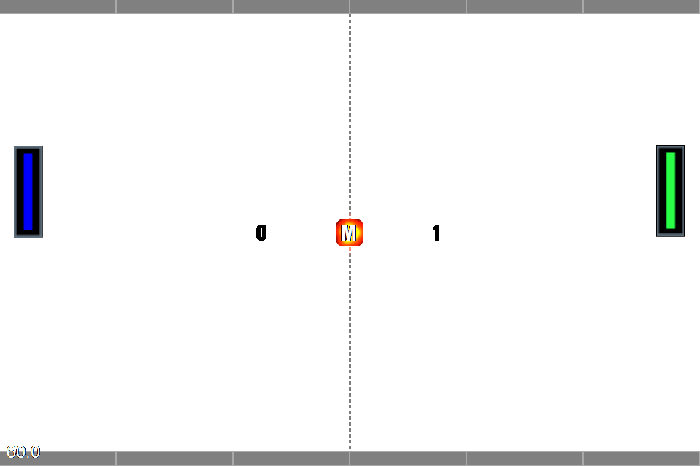# How to make a game like Pong with Felgo - Special Effects

## Special Effects

Create a new folder called particles in your qml folder. Add a FireParticle.json which will be used for the respawn animation. The particle can be created with Particle Editor Demo but for the tutorial you can just copy following code into your file.

` { "FireParticle" : { "angleVariance" : 0, "blendFuncDestination" : 1, "blendFuncSource" : 770, "duration" : 0, "emitterType" : 0, "finishColor" : "#000000", "finishColorAlpha" : 0, "finishColorVariance" : "#000000", "finishColorVarianceAlpha" : 0, "finishParticleSize" : 45, "finishParticleSizeVariance" : 10, "gravity" : "0.0, 0.0", "maxParticles" : 42, "maxRadius" : 0, "maxRadiusVariance" : 0, "minRadius" : 0, "minRadiusVariance" : 0, "particleLifespan" : 0.9000000000000000222, "particleLifespanVariance" : 0.2000000000000000111, "positionType" : 0, "radialAccelVariance" : 0, "radialAcceleration" : 0, "rotatePerSecond" : 0, "rotatePerSecondVariance" : 0, "rotation" : 0, "rotationEnd" : 0, "rotationEndVariance" : 0, "rotationStart" : 0, "rotationStartVariance" : 0, "sourcePositionVariance" : "0.0, 0.0", "speed" : 85, "speedVariance" : 2, "startColor" : "#c2401f", "startColorAlpha" : 1, "startColorVariance" : "#000000", "startColorVarianceAlpha" : 0, "startParticleSize" : 7, "startParticleSizeVariance" : 2, "tangentialAccelVariance" : 0, "tangentialAcceleration" : 0, "textureFileName" : "particleFire.png", "x" : 0, "y" : 0 }}`

Now the new particle effect needs to be added in the Level.qml after the background image and before the paddle items.

``` ...

import "particles"

...
Particle {
id: backgroundParticle
anchors.centerIn: parent
fileName: "particles/FireParticle.json"
speed: 0
}

// Player 1 is the right player
...```

The particle effect should start when the ball is restarted. Therefore, the particle needs to be started in the `reStart()` function and it will be stopped in the timer function used in Level.qml.

``` ...
Timer {
id: startTimer
interval: 1000;
onTriggered: {
ball.reStart(gameWindowAnchorItem.width/2, parent.height/2)
backgroundParticle.stop()
collisionSound.play()
}
}

// Restarts the ball
function reStart() {
// move the ball to the middle and stop it
ball.reset(gameWindowAnchorItem.width/2, parent.height/2)
// restart ball after 1s
startTimer.start()
backgroundParticle.start()
}
...```

Now a small particle effect is triggered when the ball spawns.It is very easy to add functionality. For instance, when the ball hits the wall it could increase the maximal speed or the angle of the ball hitting the paddle should be decreased. Change Ball.qml and add a collision function in the collision collider.

``` ...
property int speed: 400

// collisions with obstacles
property int collisions: 0

...

bullet: true
body.fixedRotation: true

fixture.onBeginContact: {
collisions++

if(!(collisions%5)) {
speed += collisions*5
}

var fixture = other;
var body = other.getBody();
var entity = body.target;
var collidedEntityType = entity.entityType;
var collidingType = entity.entityType;

// The ball should act a little bit different, it should not be able to toggle between top and bottom.
// Therefore, the angle needs to be calculated and adjusted with an impulse so it acts between the top and bottom border.
var normalX = contactNormal.x;
var normalY = contactNormal.y;
var localForward = circleCollider.body.linearVelocity;
var newAngle = 0.0;

if((normalX === 1) || (normalX === -1) ) {

// ATTENTION: mention that atan2 requires arguments y, x and NOT x,y!
//console.debug("atan2(y=0,x=1):", Math.atan2(0,1));
//                var currentAngle = Math.atan2(localForward.y, localForward.x);
//                currentAngle *= 180/Math.PI;
//                console.debug("currentAngle:", currentAngle, "rotation:", rotation);

// perform mirroring and calculate the new angle
localForward.x*=-1.0;
newAngle = Math.atan2(localForward.y, localForward.x);
}
// if normalY is -1 (from the ball to the target), this means the collision was pointing DOWNWARDS! mention that this is vice versa than the graphics system, with y axis pointing down not up!
// a positive forward direction means going down (the positive y axis is pointing downwards)
else if((normalY === -1 ) || (normalY === 1) ) {
// perform mirroring and calculate the new angle
localForward.y*=-1.0;
newAngle = Math.atan2(localForward.y, localForward.x);
}

// convert from rad to deg
newAngle *= 180/Math.PI;

if(Math.abs(newAngle) < 90) { // left paddle
if( newAngle < 0)
else
}
if( newAngle < 0)
else
}

if(Math.abs(newAngle) < 90) { // left paddle
if(Math.abs(newAngle) > 65) {
if( newAngle < 0)
newAngle = -65
else
newAngle = 65
}
if(Math.abs(newAngle) < 5) {
if( newAngle < 0)
newAngle = -5
else
newAngle = 5
}
}
if(Math.abs(newAngle) > 155) {
if( newAngle < 0)
newAngle = -155
else
newAngle = 155
}
if(Math.abs(newAngle) < 110) {
if( newAngle < 0)
newAngle = -110
else
newAngle = 110
}
}
// manually set the entity rotation, because it is the target and its rotation will be used for the physics body
entity.rotation = newAngle;
// ATTENTION: do NOT only set the new rotation to the entity, because the position isn't forwarded automatically!
// rather, set it for the body, which will automatically set the entity rotation to the body's
// it also must be set to the body, because otherwise the calculation by setting the linearVelocity and impulse below would not be done with the updated rotation value!
circleCollider.body.rotation = newAngle;

// this is important, otherwise the ball would get faster every time it collides with a paddle!
circleCollider.body.linearVelocity = Qt.point(0,0);

applyForwardImpulse(speed)
}
}
...```

Now, the ball increases its speed every fifth hit of an obstacle. To make the speed change even more visible you could add a new particle which spawns when the speed is increased. The new particle can be added directly to the source, because you use the fire particle effect and only change some properties. Add some more particles so it looks good and remove the speed and add a duration. Finally you have to change the blending states and the colors to make it visible on the white background.

``` ...
speed += collisions*5
speedParticle.start()
}
...
// Speed particle
Particle {
id: speedParticle
fileName: "../particles/FireParticle.json"

speed: 0
duration: 0.9
startColor: Qt.rgba(1,1,1,1);

blendFuncSource: 1
blendFuncDestination: 771

z: sprite.z-1
}
...```

Now the speed change is indicated by a small fire burst.